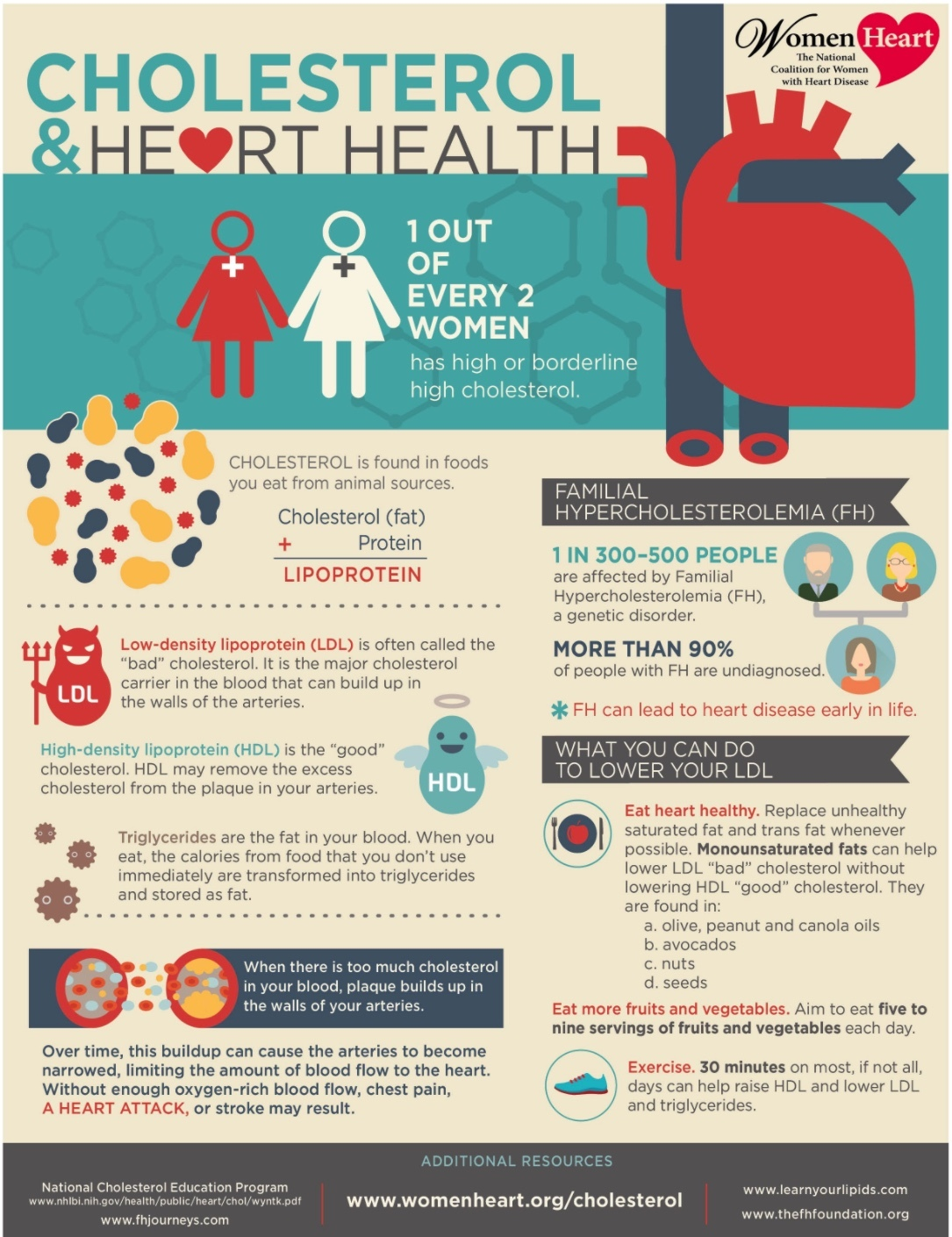## Resolution to Lifestyle

 /* styles */ No Matter The Day - It Will Be Music2YourFeet! -Denise Bryant, Founder
 table div table+table+table+table+table+table div table{width:100%;padding:0}table div table+table+table+table+table+table div table img{width:96.23%;padding:0;float:none}table div table+table+table+table+table+table div table td{width:100%;padding:0 1.88% 18px}/* styles *//* styles */

September is National Cholesterol Education Month, a good time to get your blood cholesterol checked and take steps to lower it if it is high. National Cholesterol Education Month is also a good time to learn about lipid profiles and about food and lifestyle choices that help you reach personal cholesterol goals.

What is cholesterol?

Cholesterol is a waxy, fat-like substance found in your body and many foods. Your body needs cholesterol to function normally and makes all that you need. Too much cholesterol can build up in your arteries. After a while, these deposits narrow your arteries, putting you at risk for heart disease and stroke.

How do you know if your cholesterol is high?

High cholesterol usually doesn’t have any symptoms. As a result, many people do not know that their cholesterol levels are too high. However, doctors can do a simple blood test to check your cholesterol. High cholesterol can be controlled through lifestyle changes or if it is not enough, through medications.

It’s important to check your cholesterol levels. High cholesterol is a major risk factor for heart disease, the leading cause of death in the United States.

If you have high cholesterol, what can you do to lower it?

▪ Low-fat and high-fiber food (Eat more fresh fruits, fresh vegetables, and whole grains).
▪ For adults, getting at least 2 hours and 30 minutes of moderate or 1 hour and 15 minutes of vigorous physical activity a week. For those aged 6-17, getting 1 hour or more of physical activity each day. Join Music2YourFeet virtually on our YouTube channel or at one of our outdoor dance classes (must wear your mask and practice social distancing).
▪ Maintain a healthy weight.
▪ Don’t smoke or quit if you smoke.
 ▪ Low-fat and high-fiber food (Eat more fresh fruits, fresh vegetables, and whole grains).
 ▪ For adults, getting at least 2 hours and 30 minutes of moderate or 1 hour and 15 minutes of vigorous physical activity a week. For those aged 6-17, getting 1 hour or more of physical activity each day. Join Music2YourFeet virtually on our YouTube channel or at one of our outdoor dance classes (must wear your mask and practice social distancing).
 ▪ Maintain a healthy weight.
 ▪ Don’t smoke or quit if you smoke.

National Heart Disease and Stroke Prevention Program
Since 1998, CDC has funded state health departments’ efforts to reduce the number of people with heart disease or stroke. Health departments in 41 states and the District of Columbia currently receive funding for these activities. These programs promote policy and system level changes at the state and community level to support heart-healthy and stroke-free living and working conditions.

WISEWOMAN
The WISEWOMAN program helps women with little or no health insurance reduce their risk for heart disease, stroke, and other chronic diseases. The program helps women aged 40 to 64 years quit smoking, improve their diet, and increase physical activity. WISEWOMAN also offers tests for high blood pressure, high cholesterol, and diabetes. CDC funds 21 WISEWOMAN projects in 19 states and two tribal organizations.

Lipid Standardization Program
CDC’s Environmental Health Laboratory serves as the world reference laboratory for measuring cholesterol, triglycerides, high-density lipoproteins, and low-density lipoproteins. Doctors require accurate measurements to diagnose and properly treat people with high cholesterol levels. The laboratory helps ensure the quality of about 35 million cholesterol measurements made annually in the United States.

Here's to your Safety and Health in 2020!
Denise

Source: CDC

 table div table+table+table+table+table+table+table+table+table div table{width:100%;padding:0}table div table+table+table+table+table+table+table+table+table div table img{width:96.23%;padding:0;float:none}table div table+table+table+table+table+table+table+table+table div table td{width:100%;padding:0 1.88% 18px}/* styles */table div table+table+table+table+table+table+table+table+table+table+table div table{width:100%;padding:0}table div table+table+table+table+table+table+table+table+table+table+table div table img{width:96.23%;padding:0;float:none}table div table+table+table+table+table+table+table+table+table+table+table div table td{width:100%;padding:0 1.88% 18px}/* styles */## CAROLINE PLEASANT in "For Appearances Sake"

 /* styles */ Hello M2YF Family: I hope you’re all safe and well! Things are different now, and folks are trying to do things in a new way! I’m in a Zoom Video Scene with JWHill Productions LLC, called “For Appearances Sake.” My character is Sister Gladys! This video is lighthearted! After my Scene, there is another one. This is short, too! The whole show is 30 minutes long. It will be on You Tube for a few days, if you care to take a peek! Thank you, if you do! ENJOY! Peace and Blessings, Caroline Pleasant
 table.module-14{width:99.81%;padding:0}table div table+table+table+table+table+table+table+table+table+table+table+table+table+table+table div table{width:99.81%;float:none;margin-left:auto;margin-right:auto;padding:0}table div table+table+table+table+table+table+table+table+table+table+table+table+table+table+table div table a{border:0 none;text-decoration:none}table div table+table+table+table+table+table+table+table+table+table+table+table+table+table+table div table img{width:100%!important;border:0 none;text-decoration:none}table div table+table+table+table+table+table+table+table+table+table+table+table+table+table+table div table td{width:100%;padding:0}/* styles */
 table div table+table+table+table+table+table+table+table+table+table+table+table+table+table+table+table div table{width:100%;padding:0}table div table+table+table+table+table+table+table+table+table+table+table+table+table+table+table+table div table img{width:96.23%;padding:0;float:none}table div table+table+table+table+table+table+table+table+table+table+table+table+table+table+table+table div table td{width:100%;padding:0 1.88% 18px}/* styles */## Leslie Garry is Lady Spicetable div table+table+table+table+table+table+table+table+table+table+table+table+table+table+table+table+table+table+table+table div table{width:100%;padding:0}table div table+table+table+table+table+table+table+table+table+table+table+table+table+table+table+table+table+table+table+table div table img{width:96.23%;padding:0;float:none}table div table+table+table+table+table+table+table+table+table+table+table+table+table+table+table+table+table+table+table+table div table td{width:100%;padding:0 1.88% 18px}/* styles */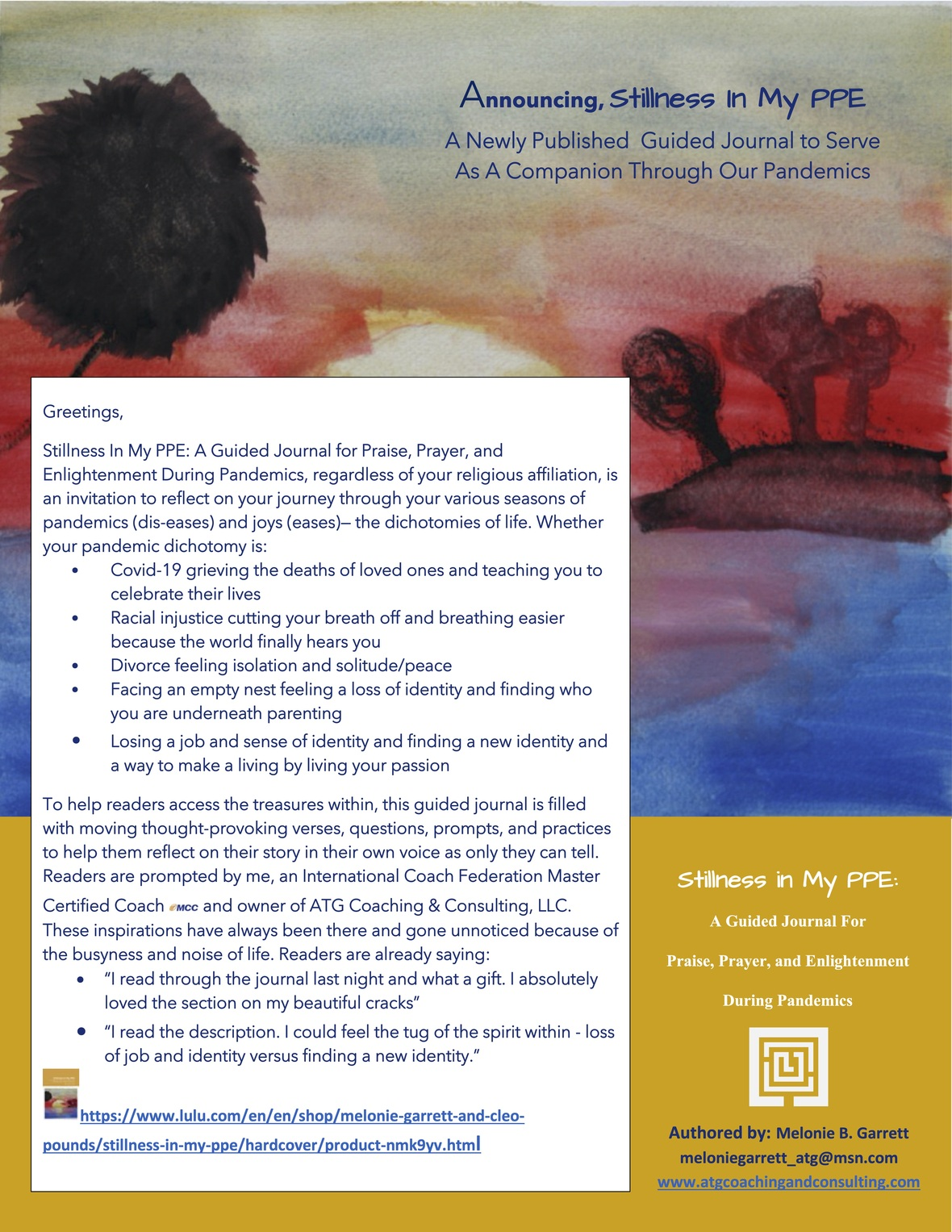/* styles */ Denise, I want to share with you and your beautiful community my recently published prompt journal, Stillness In My PPE: A Guided Journal of Praise, Prayer, and Enlightenment During Pandemics. I feel it can be a help to your dance ministry members as we navigate these turbulent times. For it was in the stillness of my own pandemic season of Covid-19 and racial injustice, this prompt journal was placed very explicitly on my heart. Hence this journal was born and I am compelled to share it with the world. For more of the backstory about my journal publication, please see my interview post on YouTube. The journal is available for purchase at Lulu.com.
 table div table+table+table+table+table+table+table+table+table+table+table+table+table+table+table+table+table+table+table+table+table+table+table div table{width:100%;padding:0}table div table+table+table+table+table+table+table+table+table+table+table+table+table+table+table+table+table+table+table+table+table+table+table div table img{width:96.23%;padding:0;float:none}table div table+table+table+table+table+table+table+table+table+table+table+table+table+table+table+table+table+table+table+table+table+table+table div table td{width:100%;padding:0 1.88% 18px}/* styles */## Denise's Corner## “The Boy” Bj, officially starting his 11th Grade year and his tenure as one of The Potomac School student representatives for the Student Diversity Leadership Conference.table div table+table+table+table+table+table+table+table+table+table+table+table+table+table+table+table+table+table+table+table+table+table+table+table+table+table+table div table{width:100%;padding:0}table div table+table+table+table+table+table+table+table+table+table+table+table+table+table+table+table+table+table+table+table+table+table+table+table+table+table+table div table img{width:96.23%;padding:0;float:none}table div table+table+table+table+table+table+table+table+table+table+table+table+table+table+table+table+table+table+table+table+table+table+table+table+table+table+table div table td{width:100%;padding:0 1.88% 18px}/* styles */Congratulations on our newest addition to our family, my great great niece living in Savannah , Ga

## Saniya Renae Letman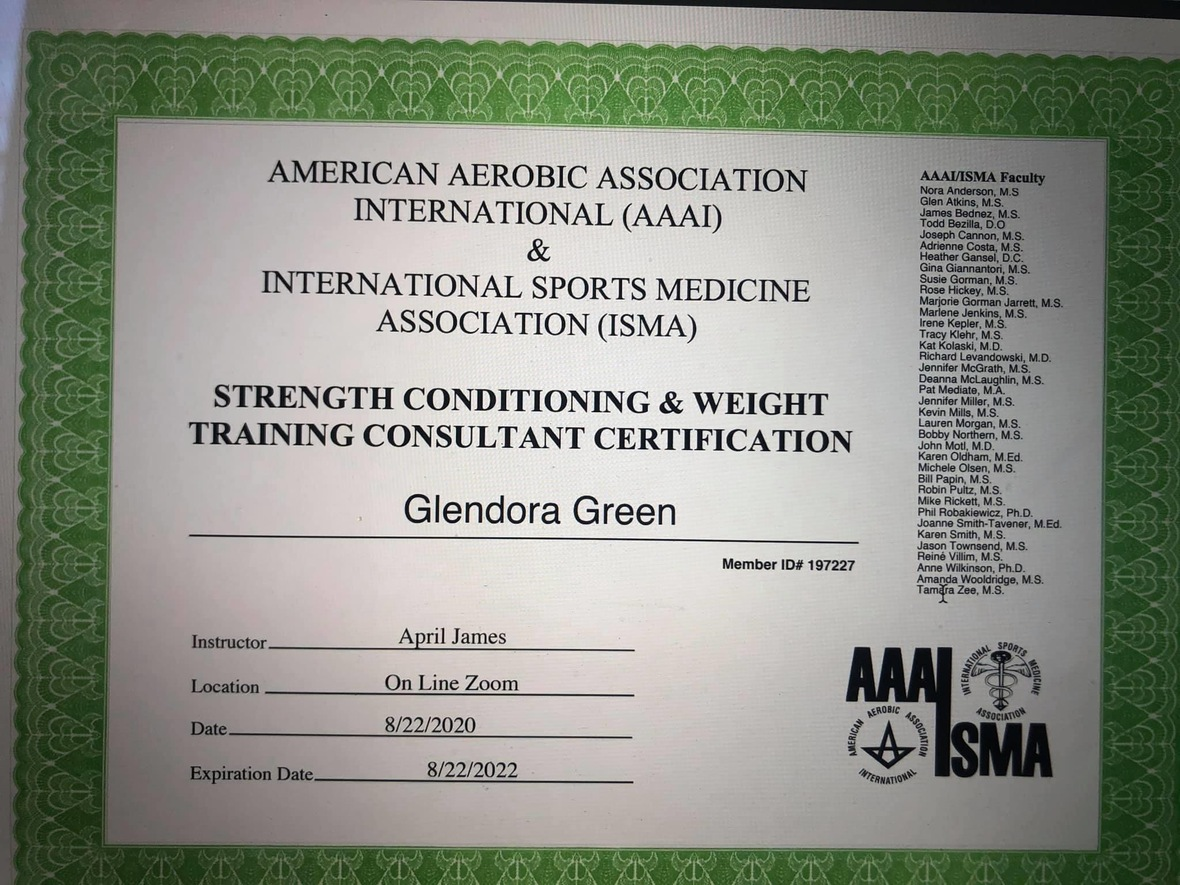## Congratulations Glendora!

 table div table+table+table+table+table+table+table+table+table+table+table+table+table+table+table+table+table+table+table+table+table+table+table+table+table+table+table+table+table+table+table div table{width:100%;padding:0}table div table+table+table+table+table+table+table+table+table+table+table+table+table+table+table+table+table+table+table+table+table+table+table+table+table+table+table+table+table+table+table div table img{width:96.23%;padding:0;float:none}table div table+table+table+table+table+table+table+table+table+table+table+table+table+table+table+table+table+table+table+table+table+table+table+table+table+table+table+table+table+table+table div table td{width:100%;padding:0 1.88% 18px}/* styles */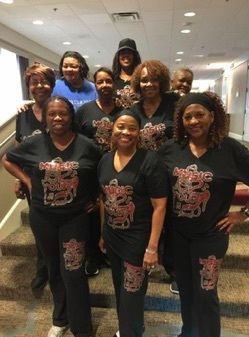Music2YourFeet Workout Suit (2 Piece)

\$40 for set, separate \$20 each,
come in black and gray only, stretch material...size from small to 2x pants and 3X shirt, may use Cash App \$seafo1 to pay, or cash, sorry no paypal and no checks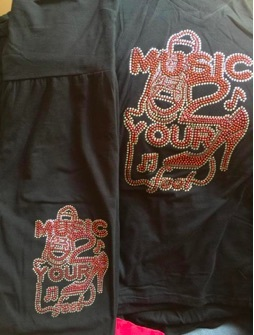Music2YourFeetWorkout Suit (pant and t-shirt)Music2YourFeet T-Shirt

\$15 each, may use Cash App \$seafo1 to pay, or cash, sorry no paypal and no checks

Please indicate the T-shirt color when ordering.
Colors available:
Green, Black, Hot Pink, Purple, Orange and Light Pink,

 table div table+table+table+table+table+table+table+table+table+table+table+table+table+table+table+table+table+table+table+table+table+table+table+table+table+table+table+table+table+table+table+table+table+table+table+table div table{width:100%;padding:0}table div table+table+table+table+table+table+table+table+table+table+table+table+table+table+table+table+table+table+table+table+table+table+table+table+table+table+table+table+table+table+table+table+table+table+table+table div table img{width:96.23%;padding:0;float:none}table div table+table+table+table+table+table+table+table+table+table+table+table+table+table+table+table+table+table+table+table+table+table+table+table+table+table+table+table+table+table+table+table+table+table+table+table div table td{width:100%;padding:0 1.88% 18px}/* styles *//* styles */ Special Birthday shout outs to my family and friends....A very Special Happy Birthday to cousin Yvette Fletcher, Happy 60th birthday, Brenda Smith, Lois Jones, Toni Coates, Gary Bonham, Adrienne Jackson, Shaunae & Nia Jackson, David Jackson, Jr. (DJ), god daughter & great niece, Mia Kidwell and all the other September babiestable div table+table+table+table+table+table+table+table+table+table+table+table+table+table+table+table+table+table+table+table+table+table+table+table+table+table+table+table+table+table+table+table+table+table+table+table+table+table+table+table div table{width:100%;padding:0}table div table+table+table+table+table+table+table+table+table+table+table+table+table+table+table+table+table+table+table+table+table+table+table+table+table+table+table+table+table+table+table+table+table+table+table+table+table+table+table+table div table img{width:96.23%;padding:0;float:none}table div table+table+table+table+table+table+table+table+table+table+table+table+table+table+table+table+table+table+table+table+table+table+table+table+table+table+table+table+table+table+table+table+table+table+table+table+table+table+table+table div table td{width:100%;padding:0 1.88% 18px}/* styles */www.music2yourfeet.com/videos.html
 /* styles */ View new line dance videos: http://www.music2yourfeet.com/videos.html
 /* styles */ Music2YourFeet visits many areas within the community, centers, parks and other events - spreading our love of line dancing. We capture every moment with video and photos. Please check out our photo gallery each month - you may find yourself in them - "Aroundtown"! View August 2020 Aroundtown Photo Gallery
 table div table+table+table+table+table+table+table+table+table+table+table+table+table+table+table+table+table+table+table+table+table+table+table+table+table+table+table+table+table+table+table+table+table+table+table+table+table+table+table+table+table+table+table+table+table+table div table{width:100%;padding:0}table div table+table+table+table+table+table+table+table+table+table+table+table+table+table+table+table+table+table+table+table+table+table+table+table+table+table+table+table+table+table+table+table+table+table+table+table+table+table+table+table+table+table+table+table+table+table div table img{width:96.23%;padding:0;float:none}table div table+table+table+table+table+table+table+table+table+table+table+table+table+table+table+table+table+table+table+table+table+table+table+table+table+table+table+table+table+table+table+table+table+table+table+table+table+table+table+table+table+table+table+table+table+table div table td{width:100%;padding:0 1.88% 18px}/* styles *//* styles */ Special Thank you To front line employees who are helping to keep us safe, we love and appreciate you.## Interested in purchasing masks, and other items, here are just a few Folks to contact, all are on FB.

Andi Johnson, Lawanda & Sonya Gross, (L&S Wear),Velvet Johnson, (velvetprettybrowneyes Johnson), Angela Smith, (PA),Dees Master Crafts, Jaqui Bain (Memphis), Tiawana Silver, Tanya Dennis, (Bling Tees)

 table div table+table+table+table+table+table+table+table+table+table+table+table+table+table+table+table+table+table+table+table+table+table+table+table+table+table+table+table+table+table+table+table+table+table+table+table+table+table+table+table+table+table+table+table+table+table+table+table+table+table+table div table{width:100%;padding:0}table div table+table+table+table+table+table+table+table+table+table+table+table+table+table+table+table+table+table+table+table+table+table+table+table+table+table+table+table+table+table+table+table+table+table+table+table+table+table+table+table+table+table+table+table+table+table+table+table+table+table+table div table img{width:96.23%;padding:0;float:none}table div table+table+table+table+table+table+table+table+table+table+table+table+table+table+table+table+table+table+table+table+table+table+table+table+table+table+table+table+table+table+table+table+table+table+table+table+table+table+table+table+table+table+table+table+table+table+table+table+table+table+table div table td{width:100%;padding:0 1.88% 18px}/* styles */table div table+table+table+table+table+table+table+table+table+table+table+table+table+table+table+table+table+table+table+table+table+table+table+table+table+table+table+table+table+table+table+table+table+table+table+table+table+table+table+table+table+table+table+table+table+table+table+table+table+table+table+table+table+table div table td,table.module-53{width:100%;padding:0}table div table+table+table+table+table+table+table+table+table+table+table+table+table+table+table+table+table+table+table+table+table+table+table+table+table+table+table+table+table+table+table+table+table+table+table+table+table+table+table+table+table+table+table+table+table+table+table+table+table+table+table+table+table+table div table{width:100%;float:none;margin-left:auto;margin-right:auto;padding:0}table div table+table+table+table+table+table+table+table+table+table+table+table+table+table+table+table+table+table+table+table+table+table+table+table+table+table+table+table+table+table+table+table+table+table+table+table+table+table+table+table+table+table+table+table+table+table+table+table+table+table+table+table+table+table div table a{border:0 none;text-decoration:none}table div table+table+table+table+table+table+table+table+table+table+table+table+table+table+table+table+table+table+table+table+table+table+table+table+table+table+table+table+table+table+table+table+table+table+table+table+table+table+table+table+table+table+table+table+table+table+table+table+table+table+table+table+table+table div table img{width:100%!important;border:0 none;text-decoration:none}/* styles */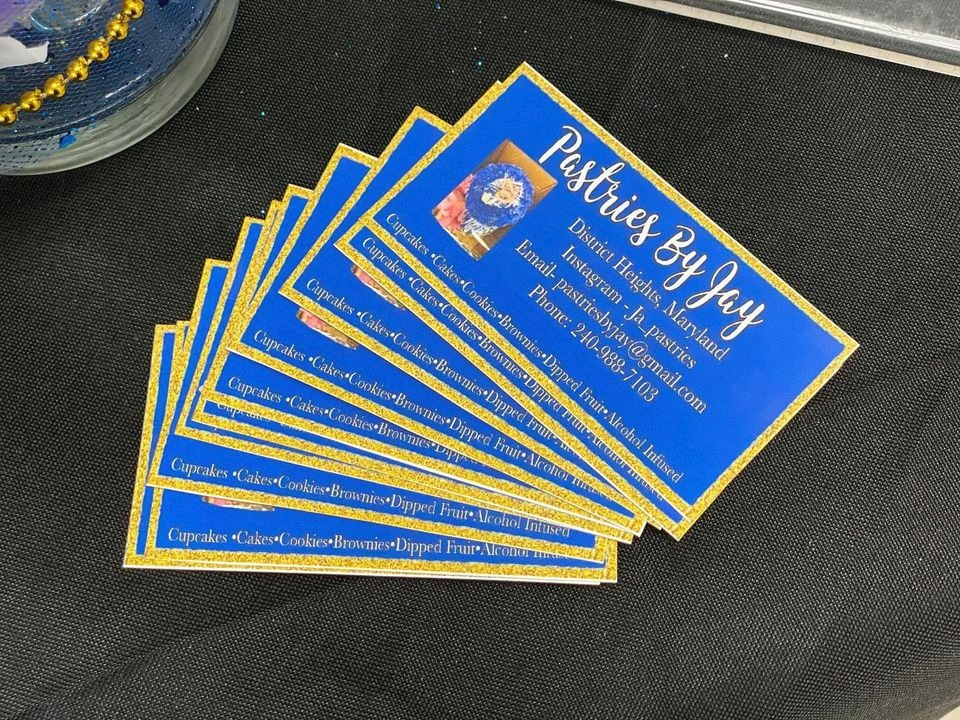table.module-55{width:61.32%;padding:0}table div table+table+table+table+table+table+table+table+table+table+table+table+table+table+table+table+table+table+table+table+table+table+table+table+table+table+table+table+table+table+table+table+table+table+table+table+table+table+table+table+table+table+table+table+table+table+table+table+table+table+table+table+table+table+table+table div table{width:61.32%;float:none;margin-left:auto;margin-right:auto;padding:0}table div table+table+table+table+table+table+table+table+table+table+table+table+table+table+table+table+table+table+table+table+table+table+table+table+table+table+table+table+table+table+table+table+table+table+table+table+table+table+table+table+table+table+table+table+table+table+table+table+table+table+table+table+table+table+table+table div table a{border:0 none;text-decoration:none}table div table+table+table+table+table+table+table+table+table+table+table+table+table+table+table+table+table+table+table+table+table+table+table+table+table+table+table+table+table+table+table+table+table+table+table+table+table+table+table+table+table+table+table+table+table+table+table+table+table+table+table+table+table+table+table+table div table img{width:100%!important;border:0 none;text-decoration:none}table div table+table+table+table+table+table+table+table+table+table+table+table+table+table+table+table+table+table+table+table+table+table+table+table+table+table+table+table+table+table+table+table+table+table+table+table+table+table+table+table+table+table+table+table+table+table+table+table+table+table+table+table+table+table+table+table div table td{width:100%;padding:0}/* styles */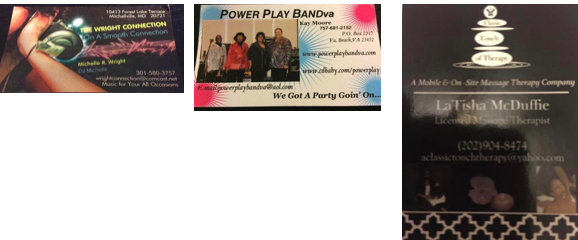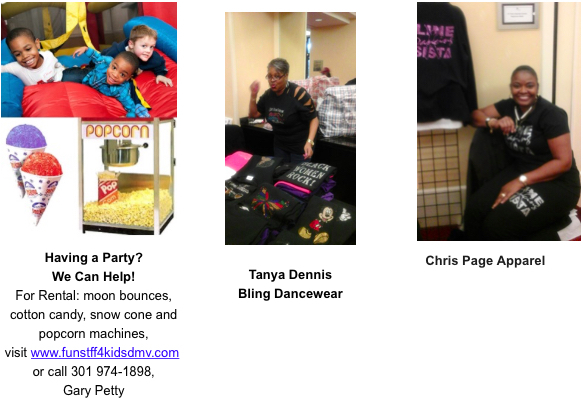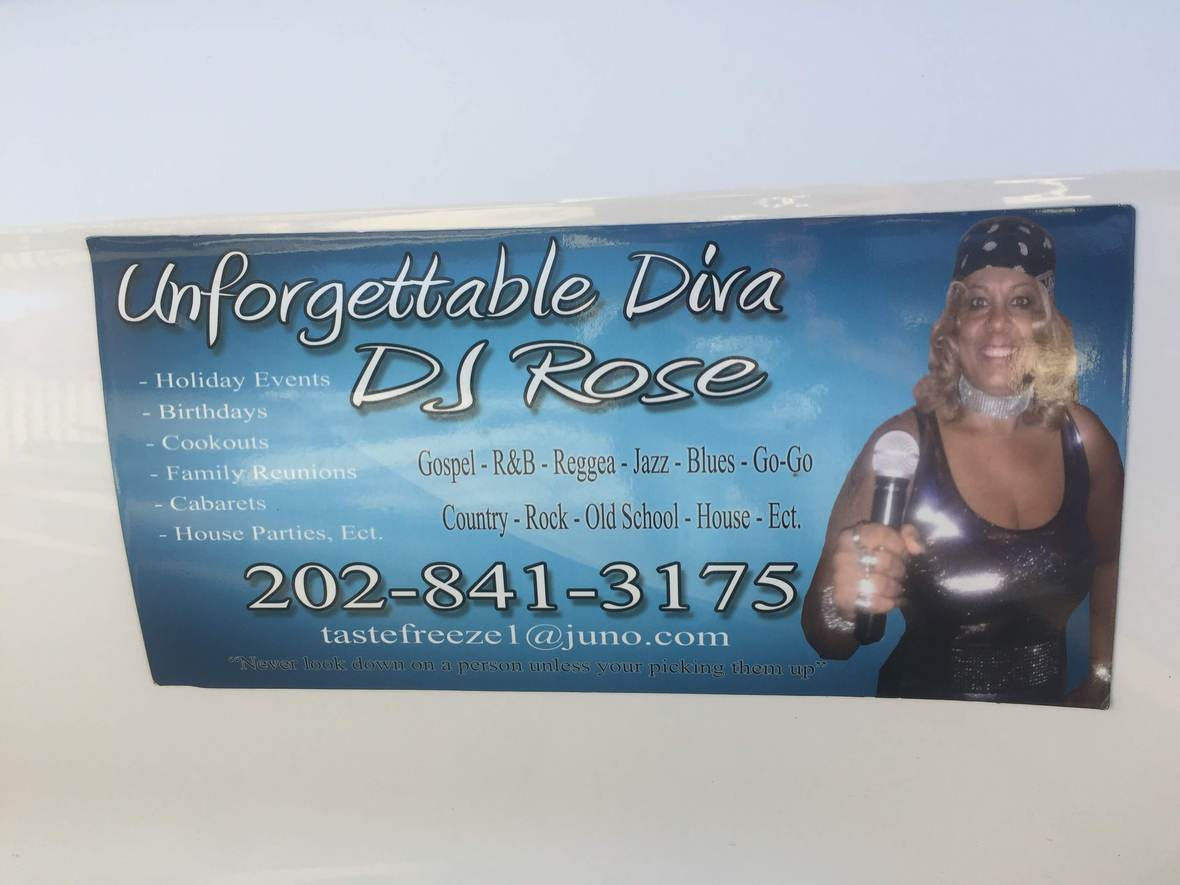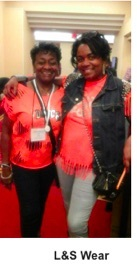# Thank you for your continued support!

## Classes and Social Events Cancelled Due to COVID-19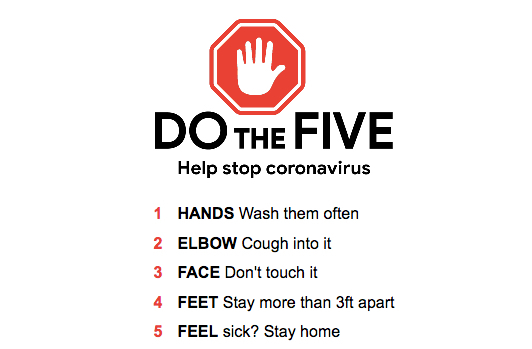Maryland and Gov. Larry Hogan (R) has declared a state of emergency, which has restricted public gatherings, businesses and government facilities, most public events have been canceled or postponed and public access to government services are increasingly restricted. With that all dance classes and social dance events are cancelled. Please check our website and Facebook page for updates.

 table div table+table+table+table+table+table+table+table+table+table+table+table+table+table+table+table+table+table+table+table+table+table+table+table+table+table+table+table+table+table+table+table+table+table+table+table+table+table+table+table+table+table+table+table+table+table+table+table+table+table+table+table+table+table+table+table+table+table+table+table+table+table+table+table+table+table+table+table+table+table div table{width:100%;padding:0}table div table+table+table+table+table+table+table+table+table+table+table+table+table+table+table+table+table+table+table+table+table+table+table+table+table+table+table+table+table+table+table+table+table+table+table+table+table+table+table+table+table+table+table+table+table+table+table+table+table+table+table+table+table+table+table+table+table+table+table+table+table+table+table+table+table+table+table+table+table+table div table img{width:96.23%;padding:0;float:none}table div table+table+table+table+table+table+table+table+table+table+table+table+table+table+table+table+table+table+table+table+table+table+table+table+table+table+table+table+table+table+table+table+table+table+table+table+table+table+table+table+table+table+table+table+table+table+table+table+table+table+table+table+table+table+table+table+table+table+table+table+table+table+table+table+table+table+table+table+table+table div table td{width:100%;padding:0 1.88% 18px}/* styles */